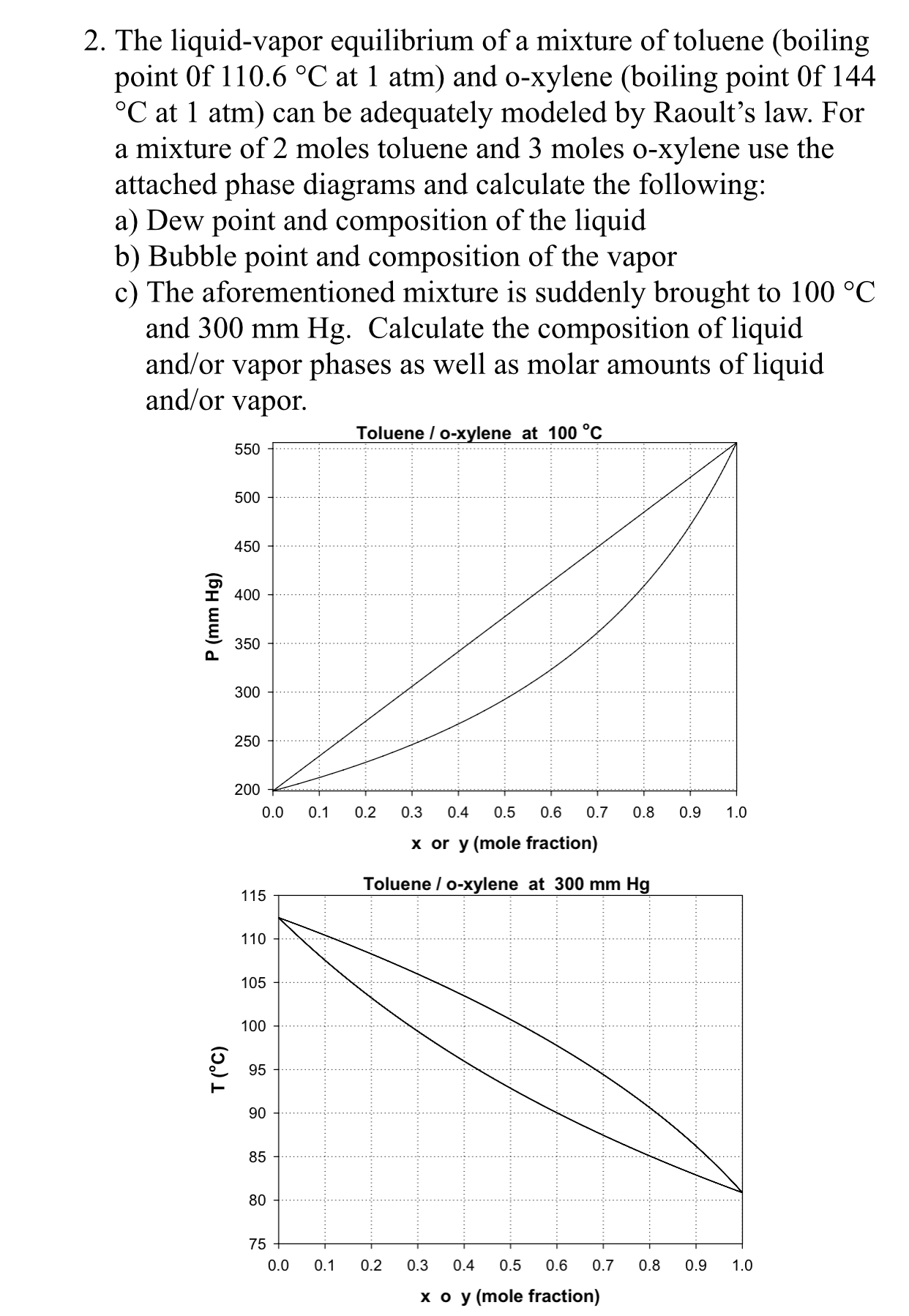# 2. The liquid-vapor equilibrium of a mixture of toluene (boilingpoint Of 110.6 °C at 1 atm) and o-xylene (boiling point Of 144C at 1 atm) can be adequately modeled by Raoult's law. Fora mixture of 2 moles toluene and 3 moles o-xylene use theattached phase diagrams and calculate the following:a) Dew point and composition of the liquidb) Bubble point and composition of the vaporc) The aforementioned mixture is suddenly brought to 100 oCand 300 mm Hg. Calculate the composition of liquidand/or vapor phases as well as molar amounts of liquidand/or vaporToluene/ o-Xylene at 100°C550500450I 4003503002502000.0 0.1 0.2 0.3 0.4 0.5 0.6 0.7 0.8 0.9 .0x or y (mole fraction)Toluene / o-xylene at 300 mm Hg115110105100CO9 95908580750.0 0.1 0.2 0.3 0.4 0.5 0.6 0.7 0.8 0.9 .0x o y (mole fraction)

Question
442 viewshelp_outlineImage Transcriptionclose2. The liquid-vapor equilibrium of a mixture of toluene (boiling point Of 110.6 °C at 1 atm) and o-xylene (boiling point Of 144 C at 1 atm) can be adequately modeled by Raoult's law. For a mixture of 2 moles toluene and 3 moles o-xylene use the attached phase diagrams and calculate the following: a) Dew point and composition of the liquid b) Bubble point and composition of the vapor c) The aforementioned mixture is suddenly brought to 100 oC and 300 mm Hg. Calculate the composition of liquid and/or vapor phases as well as molar amounts of liquid and/or vapor Toluene/ o-Xylene at 100°C 550 500 450 I 400 350 300 250 200 0.0 0.1 0.2 0.3 0.4 0.5 0.6 0.7 0.8 0.9 .0 x or y (mole fraction) Toluene / o-xylene at 300 mm Hg 115 110 105 100 CO 9 95 90 85 80 75 0.0 0.1 0.2 0.3 0.4 0.5 0.6 0.7 0.8 0.9 .0 x o y (mole fraction) fullscreen
check_circle

star
star
star
star
star
1 Rating
Step 1

A mixture of 2 moles of Toluene and 3 moles of o-xylene is given.

(a) To calculate the dew point and the liquid composition, consider the following steps:

1. Calculate the overall mole fraction for the more volatile component (xT), i.e. Toluene, in the gaseous mixture. xT = 2/2+3 = 0.4
1. On the pressure-versus-mole fraction graph, locate the point where the mole fraction is 0.4 on the x-axis.
2. From this point on the x-axis, go up till you hit the dew point curve. From here, go left till you hit the bubble point curve. Now, if you move down to the x-axis, the point where you hit the x-axis will give the liquid composition of Toluene, which is 0.2. After hitting the bubble point curve, if you continue going left to the y-axis, we get the dew point pressure as 260 mm Hg.

Do the same for calculating the dewpoint temperature on the T-vs-mole fraction graph. The dew point temperature is 104.6 0C.

(b)  To calculate the bubble point and the vapor composition, consider the following steps:

1. Calculate the overall mole fraction for the more volatile component, i.e. Toluene, in the gaseous mixture. xT = 2/5 = 0.4
1. On the Pressure-versus-mole fraction graph, locate the point where the mole fraction is 0.4 on the x-axis.
2. From this point on the x-axis, go up till you hit the bubble point curve. From here, go right till you hit the dew point curve. Now, if you move down to the x-axis, the point where you hit the x-axis will give the vapor composition of Toluene, which is 0.68. After hitting the dew point curve, if you go left to the y-axis, we get the bubble point pressure as 348 mm Hg.

Do the same for calculating the bubble point temperature on the T-versus-mole fraction graph. The bubble point temperature is 95 0C.

Step 2

(c) To calculate the composition and the molar amounts of the components of the mixture at a pressure of 300 mm Hg and temperature of 100 0C, consider the following steps.

1. Take either of the two curves and solve using the P-versus-mole fraction curve.
2. This curve is plotted at a constant temperature of 100 0C. As both the requirements are satisfied, we draw a tie line between the dew point and bubble point curve at a pressure of 300 mm Hg. The end points of the tie line give the composition of the more volatile component, i.e. Toluene, in the liquid and vapor phase as 0.3 and 0.55, respectively.

Since the mixture is binary, the sum of the mole fractions of Toluene and o-Xylene will be one. Therefore, the composition of o-xylene in the liquid phase is given as: xx = 1-0.3 = 0.7

Composition of o-xylene in the vapor phase is given as: yx = 1-0.55 = 0.45

Step 3

To calculate molar amounts of liquid (L) and vapor (V), apply overall mole balance, which is given by equation (1), where F is the total moles fed. Now, apply component balance for Toluene, which is given by equation (2), where x, y and xf are mole fractions of Toluene in the liquid phase, vapor phase and in the feed, respectively.

On solving equations (1) and (2), we get equati...

### Want to see the full answer?

See Solution

#### Want to see this answer and more?

Solutions are written by subject experts who are available 24/7. Questions are typically answered within 1 hour.*

See Solution
*Response times may vary by subject and question.
Tagged in

### Chemical Engineering